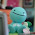## Monday, January 17, 2011

Percent - is a number out of 100
- can also be represented as a decimal or a fraction

4.2 Representing Percents

You can represent a percent by shading squares on a hundred grid. A grid that is fully shaded means 100%. Use 2 or more hundred grids to show a percent larger than 100%. If you are shading less than 1%, shade a part of one square to represent it.

4.2 Fractions, Decimals, and Percents

Percent can also be written as a fraction and decimal. To change a percent into decimal, divide the percent by 100 to get the decimal. For changing into fraction, it is easier to use a decimal because it depends on how many digits there are after the decimal.

Example : 0.157 = 157/1000.

4.3 Percent of a Number

Doubling , halving, and dividing by ten are some strategies to find the percent of a number. Another way is converting the percent into a decimal then multiply it by the number.

Example : 12½ of 50 = 0.125
0.125 x 50 = 6.25

4.4 Combining Percents

Adding percents can solve problems, Example : 5% + 7% = 12%. To calculate the increase in a number, you can either:
-Add the percent amount to the original number.
Example : 12% of 100 = 0.12x100=12, 100 + 12 = 112
-Multiply the original number by a single percent greater than 100.
Example : 112% of 100 = 1.12 x 100

1.